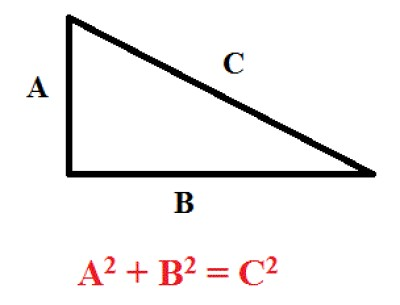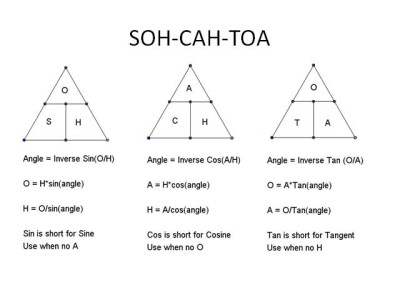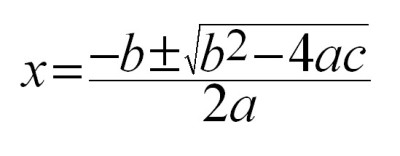List of public pages created with Protopage

# Unit A

## Photos

Labled pythagoras triangle with formula.# Unit C

## Photos

### SOH CAH TOA

This is needed in trigonometry to find sides or angles of a triangle, but when you find an angle using 2 sides you must do the cos, sin or tan to the -1. There is a separate button on the calculator to do this.### Quadratic equation

The formula for x in the quadratic formula. The letters in the equation are given in the question with what a, b and c is for the formula.